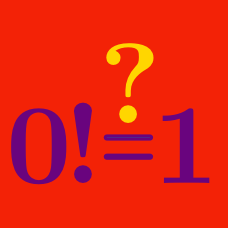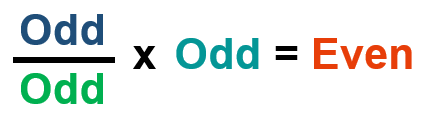Number Theory

# Number Theory Common Misconceptions: Level 1 Challenges

$\large \text{Is } 0 \text{ even or odd?}$

True or False:

$\huge{\color{#D61F06}{3} \text{ is a multiple of } \color{#D61F06}{3}.}$

Which of these is not a property of all even and odd numbers?Can an odd number, divided by another odd number, times another odd number ever equal an even number?

If "yes," then find three numbers that work. If "no," then why not?

Clarification: The three odd numbers can be different numbers.

True or False?

${\color{#3D99F6}{0} \text{ is a multiple of } \color{#D61F06}{3}.}$

×